Courses

# Harmonics in Induction Machines Electrical Engineering (EE) Notes | EduRev

## Electrical Engineering (EE) : Harmonics in Induction Machines Electrical Engineering (EE) Notes | EduRev

The document Harmonics in Induction Machines Electrical Engineering (EE) Notes | EduRev is a part of the Electrical Engineering (EE) Course Electrical Machines.
All you need of Electrical Engineering (EE) at this link: Electrical Engineering (EE)

Harmonics in Induction Machines

In attempting to understand the performance of an induction machine, we consider that the air-gap ﬂux wave is purely sinusoidal. It is from that assumption the analysis of induced emf, sinusoidal currents, the expressions for generated torque etc. proceed. In practice, there are deviations from this idealistic picture.

Time Harmonics

The ﬁrst non-ideality is the presence of harmonics in the input supply given to the three phase machine. The source may contain 3rd, 5th, 7th. . . harmonics. Note that due to the symmetry of the waveform (f (t) = −f (t + T /2), where T is the period of the supply sine waveform, even ordered harmonics cannot exist. Let the R phase supply voltage be given by the expression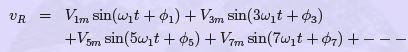(25)

Being a balanced three phase supply, we know that the waveforms of vY and vB are 120 and 240 shifted from vrespectively. It is further well known that if a waveform is shifted by φ degrees, its harmonics are shifted by nφ degrees, where n is the order of the harmonic.
Thus the expressions for vY and vwould be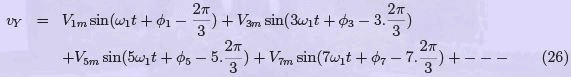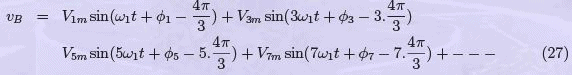If we consider the third harmonic components of the three phase waveforms, and if vx3(t) is the third harmonic of phase x, we can see that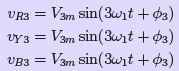(28)

Therefore, all the three third harmonics are in phase. In a STAR connected system with isolated neutral, these voltages cannot cause any current ﬂow since all three terminals are equal in potential. If the neutral point is connected to some point, then then current can ﬂow through the neutral connection. Such a connection is however rare in induction machines.
The machine is therefore an open circuit to third harmonics. In fact, one can see that any harmonic whose order is a multiple of three, i.e., the triplen harmonics, as they are called, will face an identical situation. Since the machine is an open circuit to triplen harmonics in the excitation voltage, these do not have eﬀect on the machine.

Let us now consider the ﬁfth harmonic. From the equations above, one can see that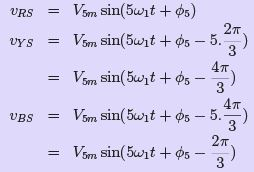(29)

From eqns. 29 we see that the ﬁfth harmonic of the excitation forms a negative sequence system — B phase lags R by 120 and Y phase lags R by 120.

The MMF caused by a negative sequence excitation causes backward revolving ﬂux pattern (compared to the direction of the fundamental). The torque which it generates will act as an opposing torque to that generated by the fundamental.

Looking at the seventh harmonic, we can see that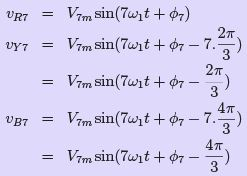(30)

From eqns. 30, it is evident that the seventh harmonic components of the excitation form a positive sequence system. The torque produced by these currents will therefore be additive with respect to the fundamental component’s torque.

The actual eﬀect of these harmonics on the induction machine would depend on the reactance of the machine since at high frequencies, it is the reactance component that dominates the inductance. Excitation voltage waveforms with considerable harmonic content may result when induction machines are controlled through inverters. Apart from the eﬀects on torque, these harmonics cause considerable heating in the machine and are hence a cause for concern. These harmonics are called time harmonics since they are generated by a source that varies non-sinusoidally in time.

Space Harmonics

Apart from this, there is another kind of harmonic generated in machines called space harmonic. To understand that this behaviour, it is necessary to consider MMF/ﬂux production in the machine. It may not be out of place to recall once again that in all our foregoing analysis we have assumed that both air-gap mmf and ﬂux are sinusoidally distributed in space.

Let us consider a single full-pitched coil excited by a sinusoidal voltage. The current ﬂowing through it is sinusoidal and hence the time variation of the mmf produced by it is sinusoidal. But if we travel around the span of the coil, the MMF variation that we would encounter is square. It is the amplitude of this square wave that varies sinusoidally in time.

The behaviour is depicted diagrammatically in ﬁg. 36.

Let one more coil be connected in series to this, which is spatially displaced by the slot angle. This is shown in ﬁg. 37. The same current passes through both and hence the mmf pattern generated by both would vary in tandem. However, they will be displaced by a slot angle as far as spatial distribution is concerned. The resulting situation is also shown in ﬁg. 37 at a particular time instant. It can be seen that the resultant mmf waveform a non-sinusoidal function of the space angle θ. The harmonics are functions of the space angle.

These are called space harmonics. Let us consider the eﬀects of these.
Let the net ﬂux waveform as a function of angle at an instant of time when unit current ﬂows in the coils be described by f (θ). Clearly f (θ) is a periodic function of θ with a period equal to 2π. Therefore one may express this as a fourier series. If fA(θ) is the distribution function for phase A,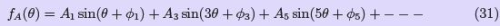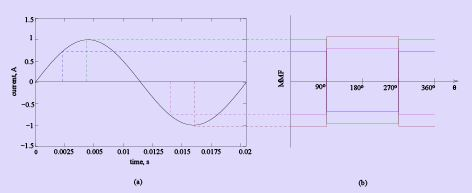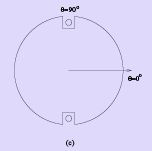Figure 36: Coil MMF with sinusoidal excitation

The distribution functions for phases B & C will be displaced from that of A phase by 120◦ and 240 respectively and hence are given by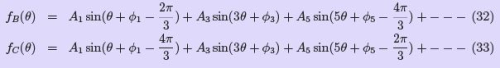Note that we have written these at a given instant of time when unit current ﬂows. We know that this current variation is sinusoidal in time. Considering the ﬁfth harmonic, let the resultant ﬁfth harmonic variation is given by,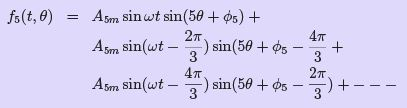(34)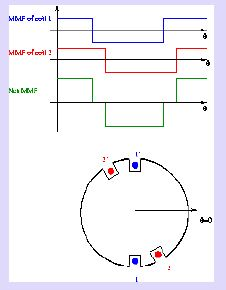Figure 37: MMF in a distributed winding in two slots

Upon simpliﬁcation, we get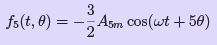(35)

Consider the behaviour of this function. At t=0, the function a value of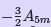at θ =0. Now let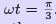At this instant, we ﬁnd that the function reaches a valueat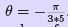In other words the function f5 (t, θ) has shifted by an angle which is a ﬁfth of the value of ωt, in the negative direction. The ﬁfth harmonic therefore rotates opposite to the direction of the fundamental at a speed which is one-ﬁfth of the fundamental.

Similarly, if we consider the seventh harmonic, it can be shown that the resultant distribution is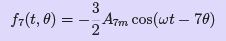(36)

By similar arguments as above we conclude that the seventh space harmonic rotates in the same direction as that of the fundamental at one seventh the speed. In general , we may have harmonics of the order h = 6n±1, where n is an integer greater than or equal to 1. Harmonics orders generated by the addition operation move in the same direction as the fundamental and those generated by the subtraction operation move in the opposite direction. The speed of rotation is ω1/h, where ω1, is the synchronous speed of the fundamental.

The space harmonics, it may be noticed are a result of non-sinusoidal distribution of the coils in the machine and slotting. These have their eﬀects on the speed torque current of the machine. An example speed-torque characteristic of an induction machine is compared with its ideal characteristic in ﬁg. 38. The eﬀect of 5th , 7th , 11th and 13th harmonics have been considered. It can be seen that these harmonics result in kinks in the speed-torque characteristic near starting region.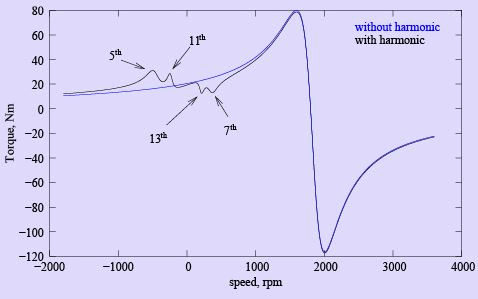Figure 38: Ideal and actual speed-torque curves

To understand the eﬀect of these kinks, consider ﬁg. 39, which shows the same speed torque characteristic in the motoring region. A load characteristic is also shown, which intersects the machine characteristic at various points. Note that point 1 is stable and hence the machine would have a tendency to operate there, though the intended operating point might be point 5. This tendency is referred to as craw ling. A momentary reduction in load torque conditions might accelerate the machine to print 2, which is unstable. The operating point would then settle down at 3. The intended operating point may be reached if favourable torque variations are there.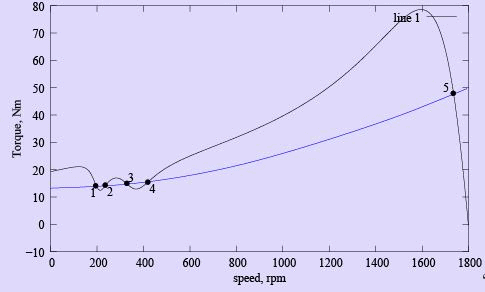Figure 39: Eﬀect of harmonics on loaded machine

Offer running on EduRev: Apply code STAYHOME200 to get INR 200 off on our premium plan EduRev Infinity!

61 docs|20 tests

,

,

,

,

,

,

,

,

,

,

,

,

,

,

,

,

,

,

,

,

,

;